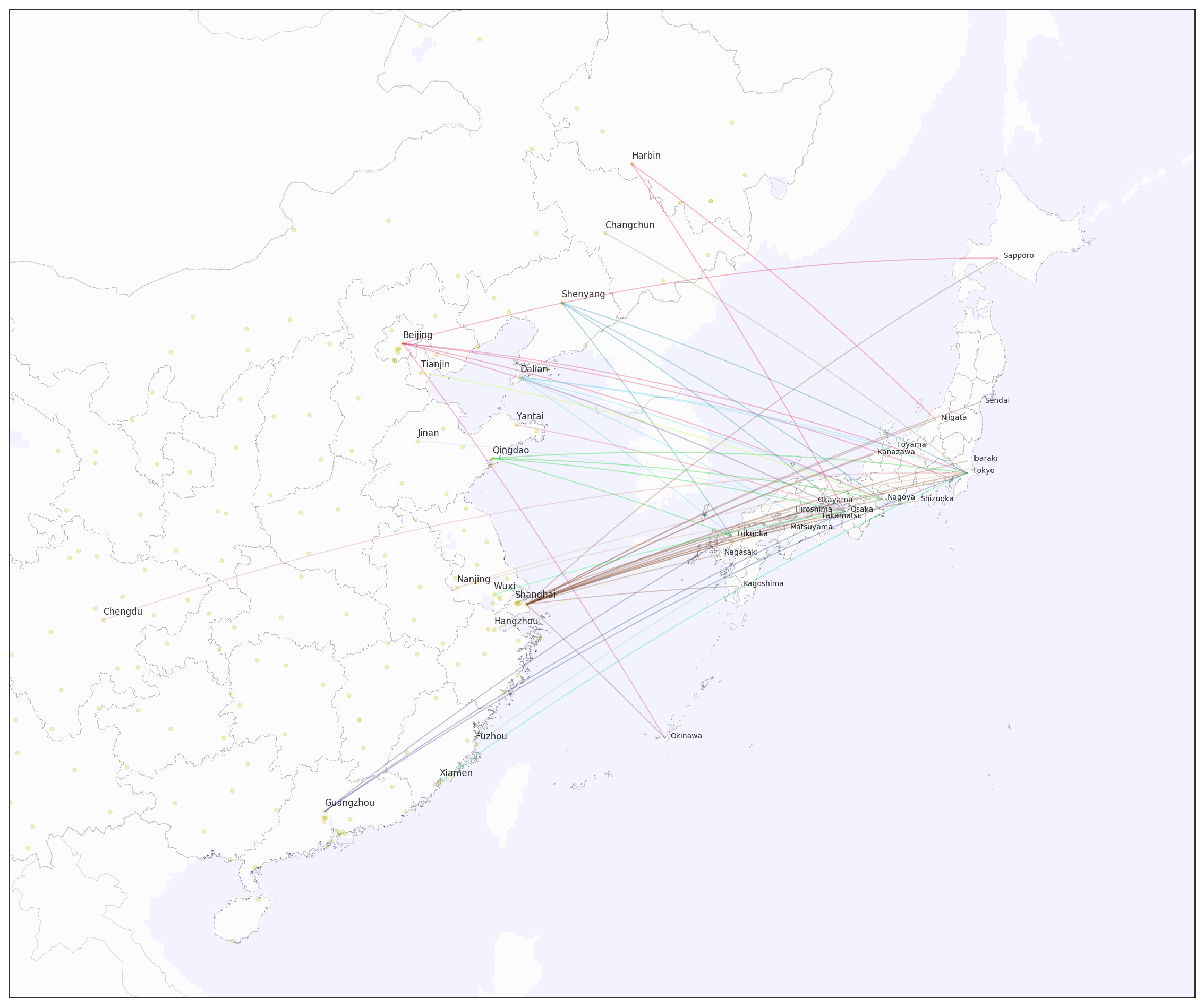Open Flights 官网提供的都是独立文本，形如 airports.dat, airlines.dat, routes.dat，好在 Data Quest 提供了一个打包的 .db 文件，我选择直接使用这一数据源。

``````import sqlite3
import pandas as pd
import numpy as np
``````
``````from mpl_toolkits.basemap import Basemap
import matplotlib.pyplot as plt
``````

• Basemap 需自行安装
• PyPI 内的 basemap 版本较老，可去 Basemap Github Repo 安装新版
• Basemap 依赖 GEOS，虽然源码附带了 geos-3.3.3，但需手工编译
• 我选择切到 Homebrew，安装 geos-3.6.2
• 之后返回仓库，再据章安装 Basemap

``````%matplotlib inline
%config InlineBackend.figure_format = 'retina'
``````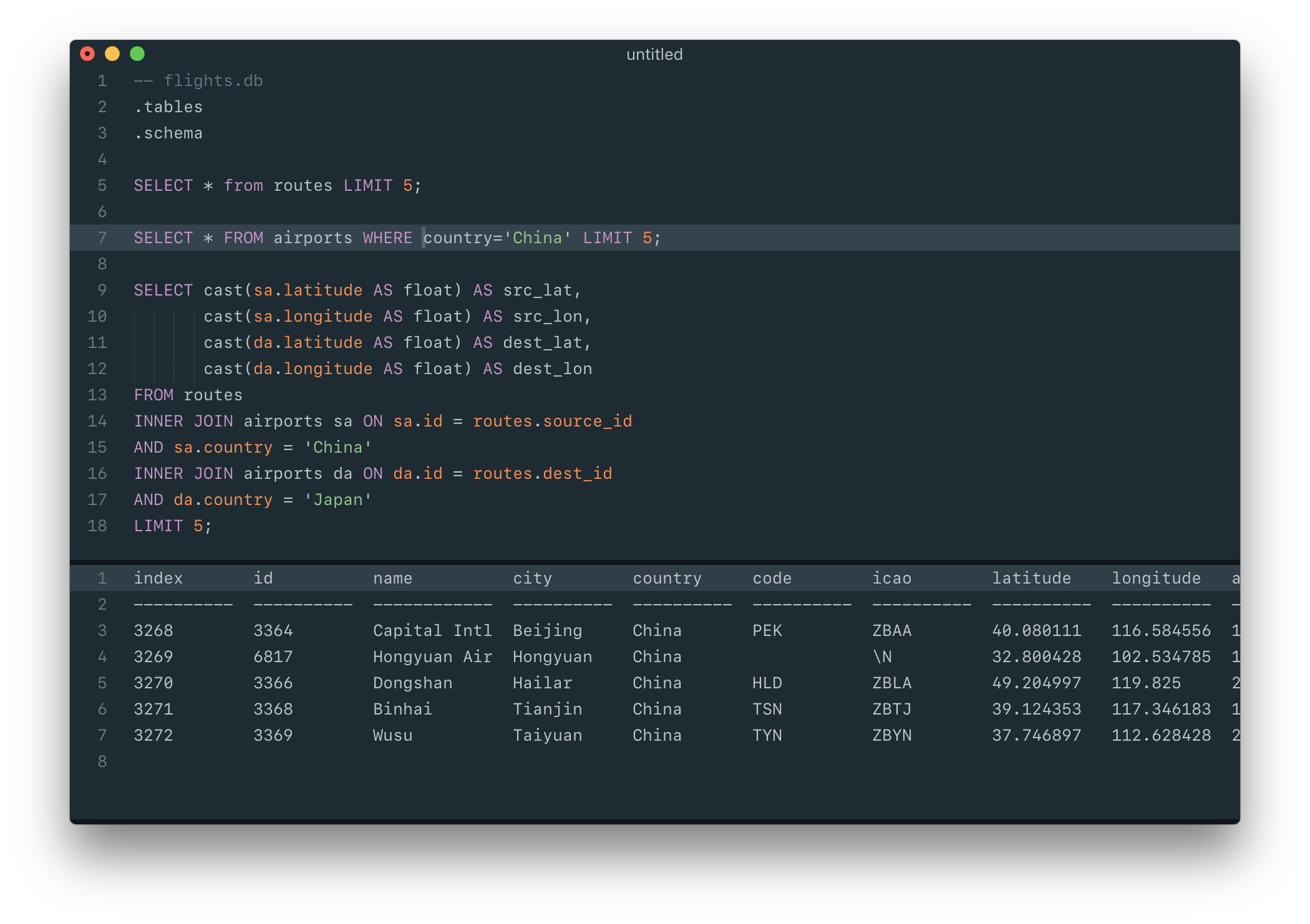``````db = sqlite3.connect('../py/data/flights.db')

"""
SELECT DISTINCT cast(latitude AS float) AS lat,
cast(longitude AS float) lon
FROM airports
WHERE country = 'China';
""",
db
)

"""
SELECT DISTINCT cast(sa.latitude AS float) AS src_lat,
cast(sa.longitude AS float) AS src_lon,
cast(da.latitude AS float) AS dest_lat,
cast(da.longitude AS float) AS dest_lon,
sa.city as src_city,
da.city as dest_city
FROM routes
INNER JOIN airports sa ON sa.id = routes.source_id
AND sa.country = 'China'
INNER JOIN airports da ON da.id = routes.dest_id
AND da.country = 'Japan';
""",
db
)
``````

1. 中国境内的所有机场经纬度
2. 涉及中日航线的两国机场经纬度
``````airports.head()
``````
lat lon
0 40.080111 116.584556
1 32.800428 102.534785
2 49.204997 119.825000
3 39.124353 117.346183
4 37.746897 112.628428
``````routes.head()
``````
src_lat src_lon dest_lat dest_lon src_city dest_city
0 40.080111 116.584556 35.764722 140.386389 Beijing Tokyo
1 40.080111 116.584556 42.775200 141.692283 Beijing Sapporo
2 40.080111 116.584556 35.552258 139.779694 Beijing Tokyo
3 40.080111 116.584556 26.195814 127.645869 Beijing Okinawa
4 40.080111 116.584556 34.858414 136.805408 Beijing Nagoya

• Projection
• 地图呈现区域
• 细节配置

``````fig, ax = plt.subplots(figsize=(20, 20))

map = Basemap(
projection = 'merc',
llcrnrlat=16,
llcrnrlon=100,
urcrnrlat=50,
urcrnrlon=150,
resolution='l'
)

map.drawcountries(color='#cccccc')
``````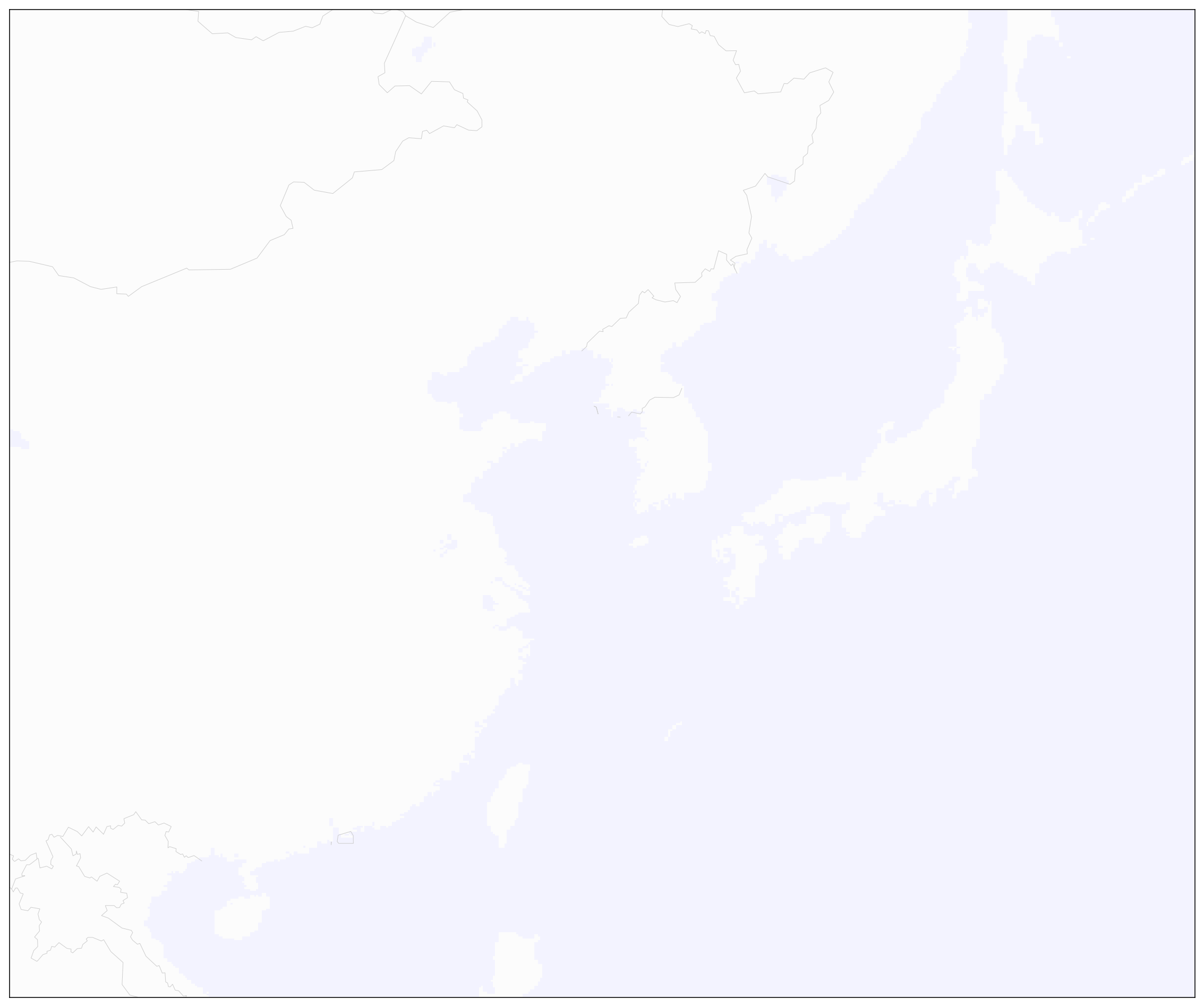## Map Projection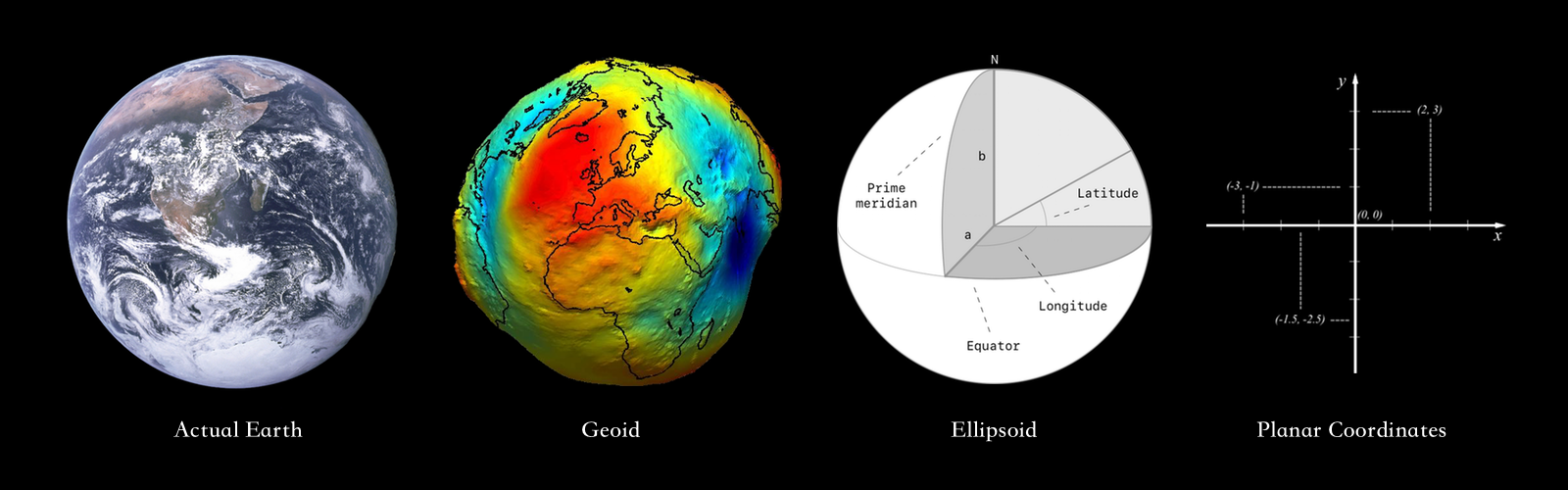• Area 面积
• Shape 形状
• Direction 方向
• Bearing 方位（注意区别 Direction）
• Distance 距离
• Scale 缩放比例

Mercator 地图的优势，就是随便在地图上的两点间画一条直线，它就是一条 Rhumb Line。它虽不是地表两点间最短路线（Great Circle），但与经线的角度固定，船不用一直变向，虽然有点绕远，可导航省事。而如果走 Great Circle，距离虽短了，可船就要时刻不停的变向，风险和操作难度都大增，而且当时经度之战刚刚开始，钟表法、月距法都还在摸索之中。### Projection 的选择

``````chn = map.readshapefile('../shapefile/CHN_adm_shp/CHN_adm1', 'chn', color='#555555', linewidth=0.1, ax=ax)
fig
````````````x, y = map(list(airports['lon']),
list(airports['lat']))

map.scatter(
x,
y,
50,
marker='.',
color='y',
alpha=0.2,
ax=ax
)

fig
``````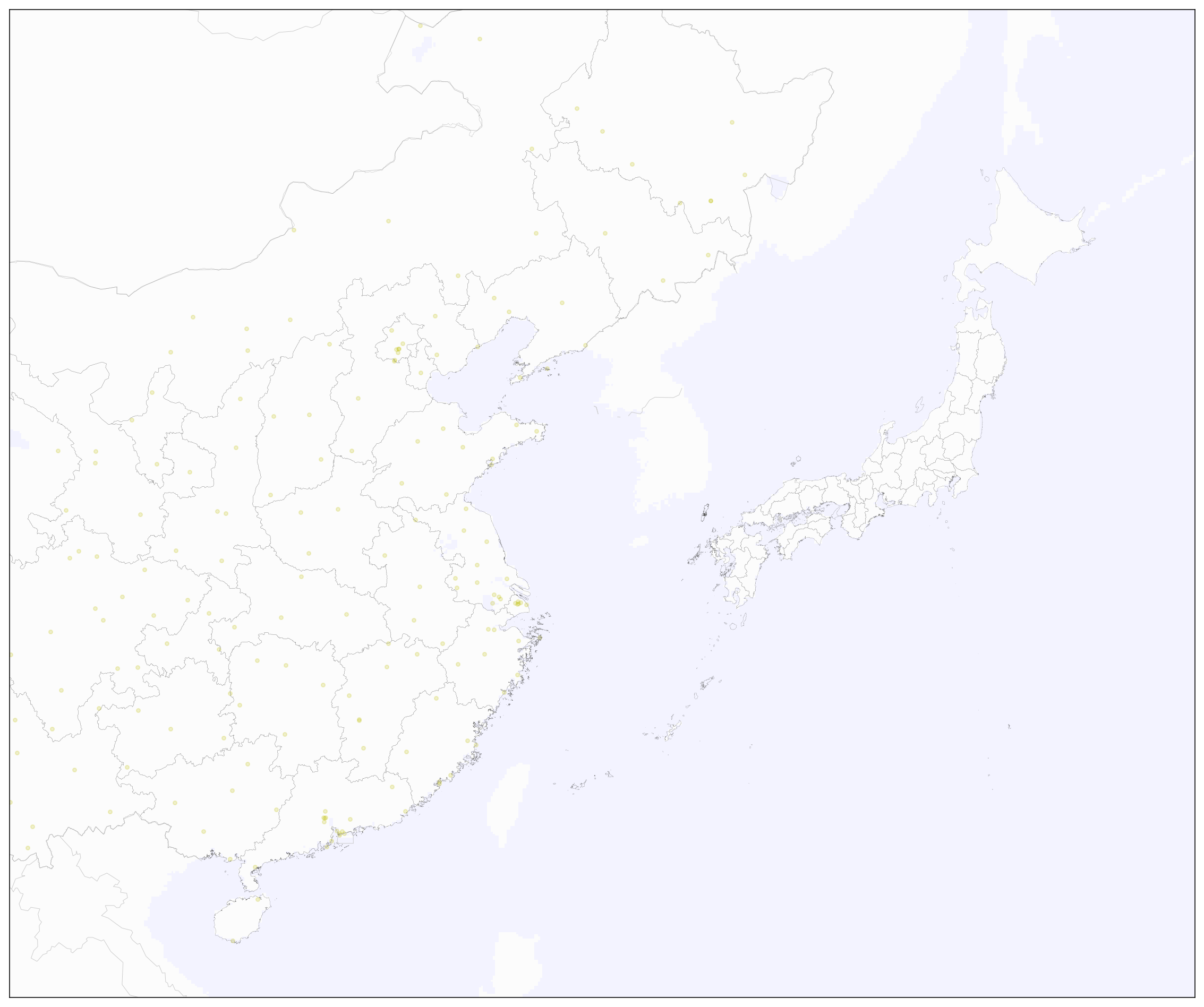``````grouped = routes.groupby('src_city')
src_city_list = grouped.groups.keys()

colors_length = np.random.random((len(src_city_list), 3))
colors = dict([(city, color) for city, color in zip(src_city_list, colors_length)])

for key, group in grouped:
for index, row in group.iterrows():
map.drawgreatcircle(
row['src_lon'],
row['src_lat'],
row['dest_lon'],
row['dest_lat'],
linewidth=1,
c=colors[key],
alpha=0.3,
ax=ax
)

fig
``````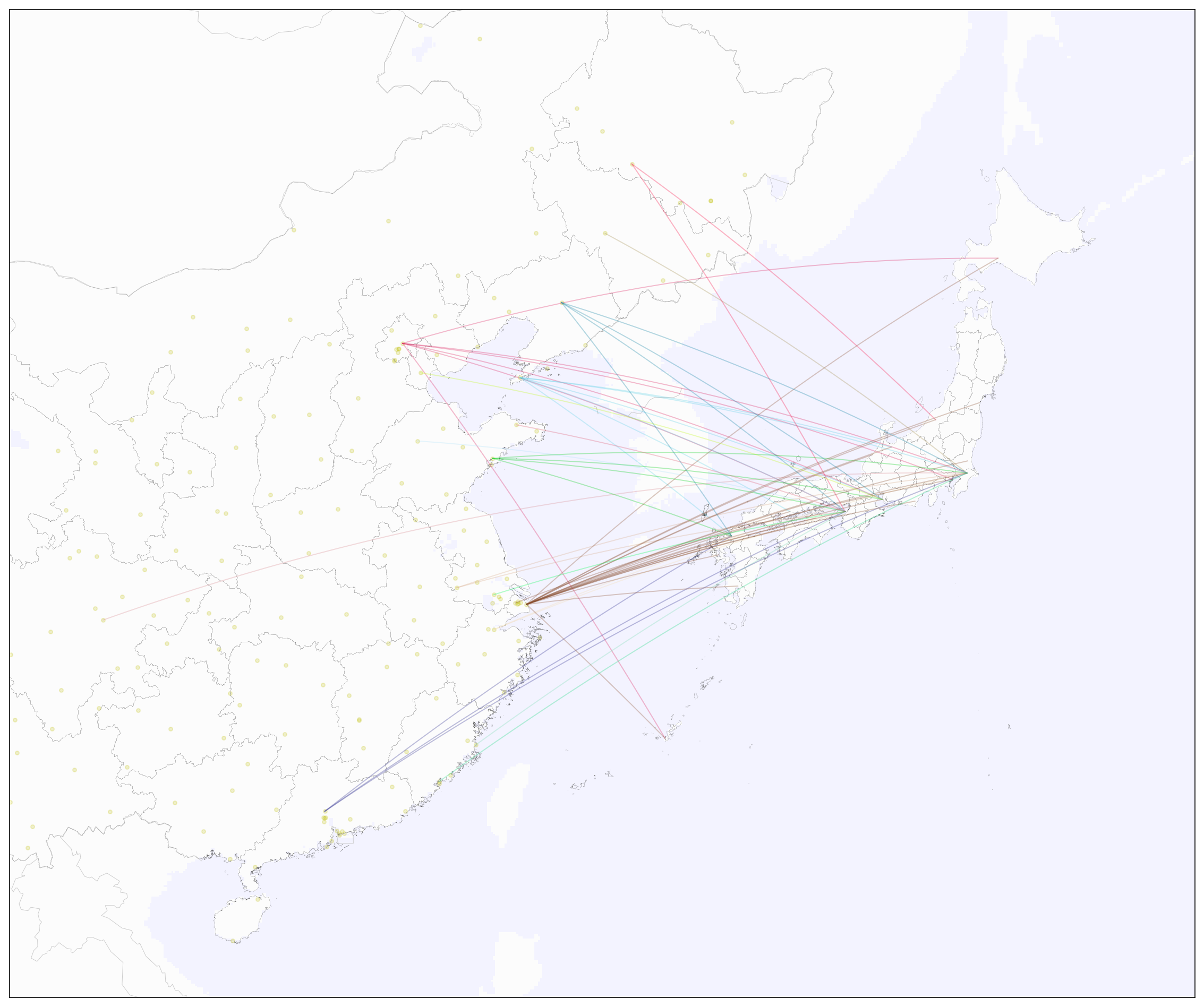``````src_labels = routes.loc[:, ['src_lat', 'src_lon', 'src_city']]
src_labels.drop_duplicates(subset=['src_city'], keep='first', inplace=True)
src_labels.reset_index(drop=True, inplace=True)

Xs, Ys = map(list(src_labels['src_lon']), list(src_labels['src_lat']))

for i, (x, y) in enumerate(zip(Xs, Ys)):
ax.annotate(str(src_labels['src_city'][i]), (x,y), xytext=(0, 5), textcoords='offset points', alpha=0.8, size='small')

fig
``````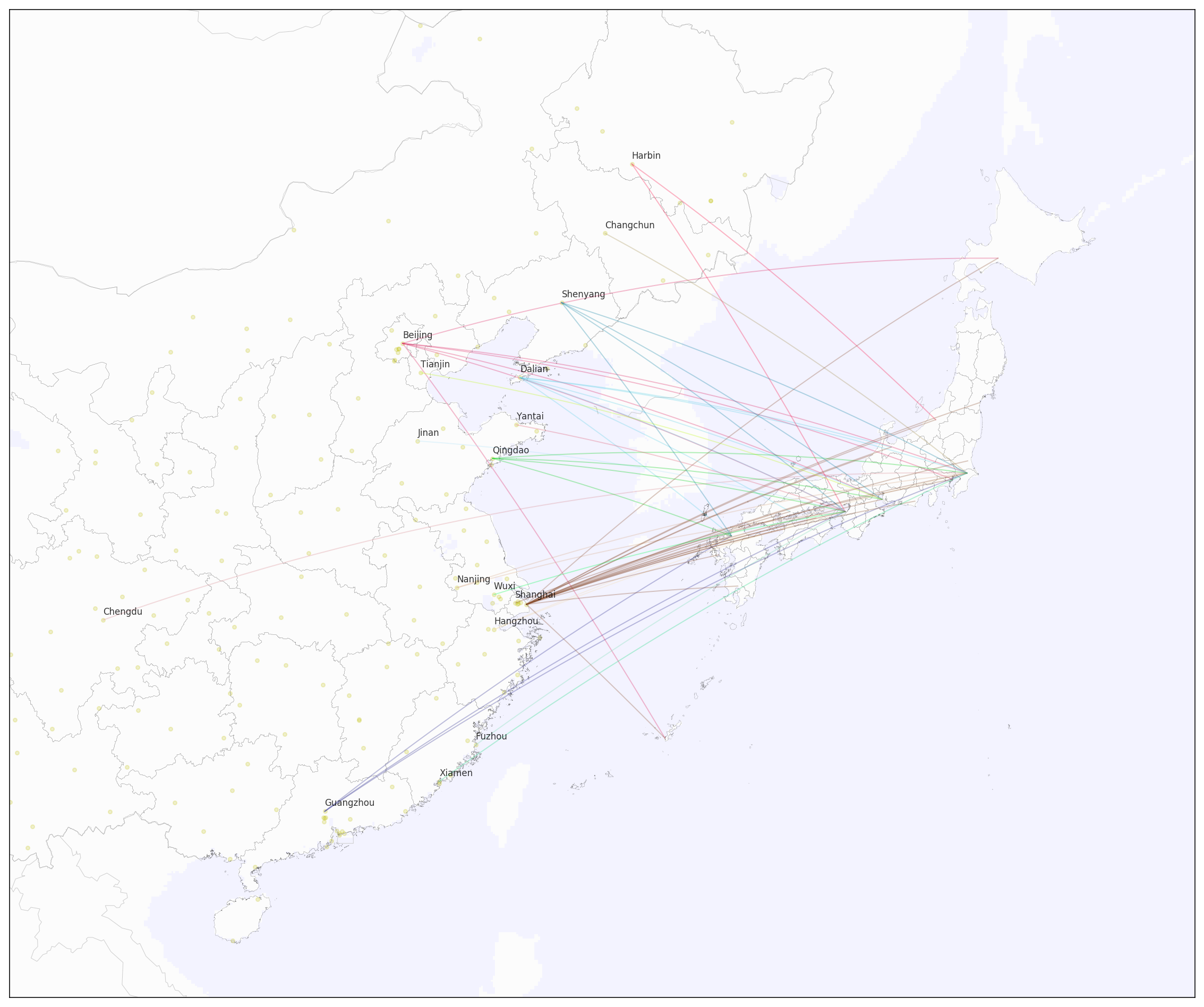``````dest_labels = routes.loc[:, ['dest_lat', 'dest_lon', 'dest_city']]
dest_labels.drop_duplicates(subset=['dest_city'], keep='first', inplace=True)
dest_labels.reset_index(drop=True, inplace=True)

Xd, Yd = map(list(dest_labels['dest_lon']), list(dest_labels['dest_lat']))

for i, (x, y) in enumerate(zip(Xd, Yd)):
ax.annotate(str(dest_labels['dest_city'][i]), (x,y), xytext=(5, 0), textcoords='offset points', alpha=0.8, size='x-small')

fig
``````